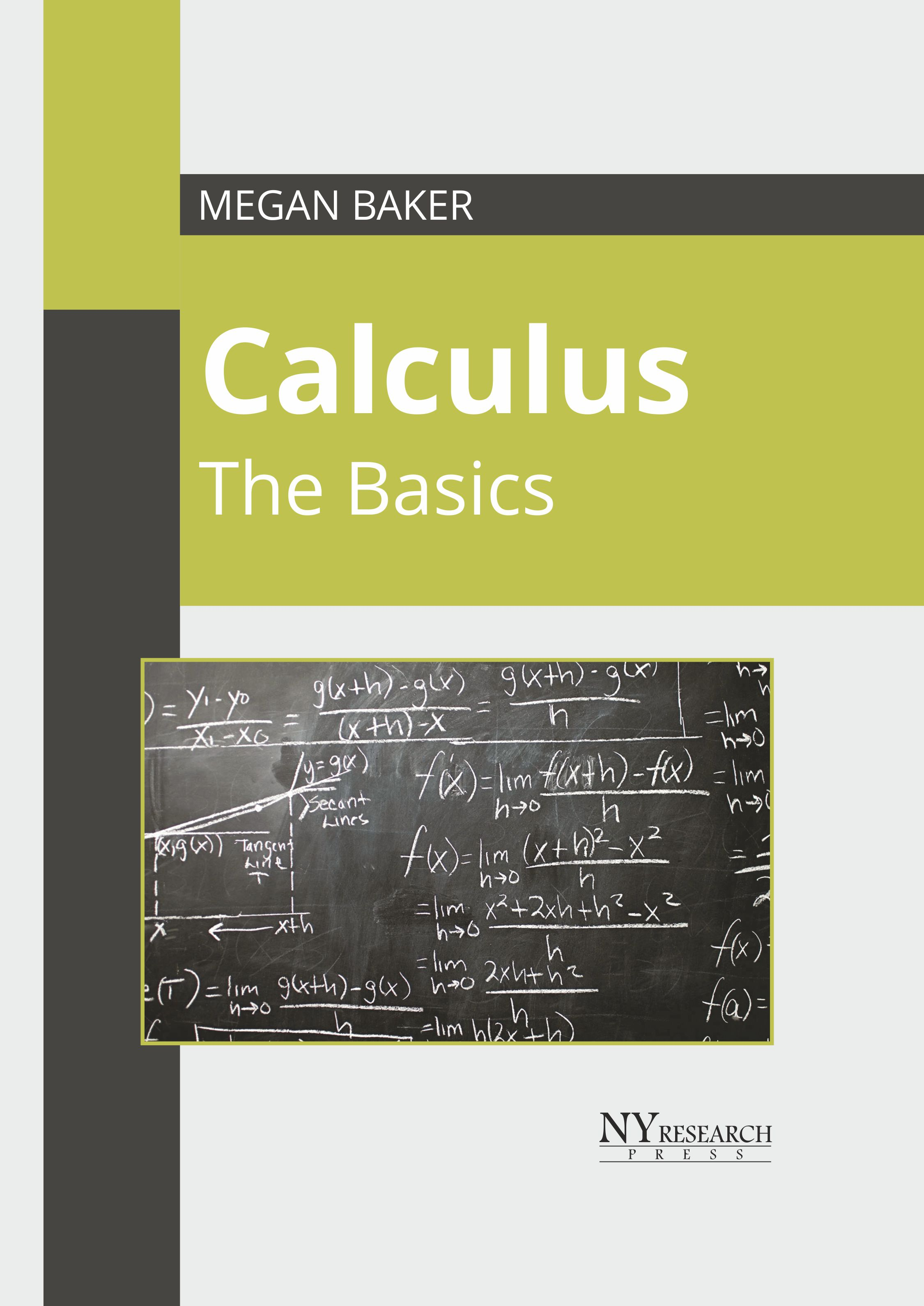BROWSE BY

#### Author

Results 1 - 2 of 2Calculus: The Basics Author : Megan Baker Subject : Chemical Engineering ISBN :9781632388254 The mathematical study of continuous change is known as calculus. There are two major divisions of calculus, known as differential calculus and integral calculus. The instantaneous rates of change and Read MoreCalculus: The Basics Author : Megan Baker Subject : Chemical Engineering ISBN :9781632388773 The mathematical study of continuous change is known as calculus. There are two major divisions of calculus, known as differential calculus and integral calculus. The instantaneous rates of change and Read More
Results 1 - 2 of 2